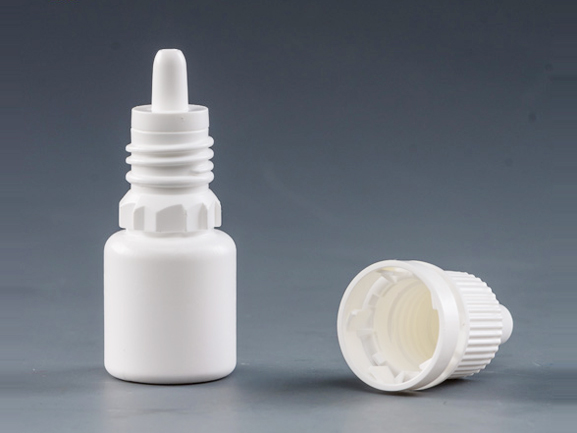# Three factors influencing the mean weight of drops dispensing

Jan 30 , 2020

The application of eye drops from flexible dropper bottles fitted with different types of dropper tips is associated with the high variability of eye drop weights. The aim of this report was to investigate the simultaneous effect of three factors influencing the mean weight of drops dispensing from two plastic dropper tips.Using a designed experiment , the effect of the concentration of benzalkonium chloride solutions  in the range of 0-0.02%, the dispensing angle from 90 degrees to 30 degrees from horizontal, and the residual volume of liquid in the dropper bottle from 4 to 10 mL on the mean drop weights were examined. The significant effect of the increase in BAC concentration resulted in a linear decrease in drop weights for both of the dropper tips investigated. The significant effect of the dispensing angle was influenced by the dropper tip design. For the dropper tip A, the effect of the dropper tip tilt was described by the quadratic equation with a minimum, which corresponded to the dispensing angle equal to that of 48 degrees from horizontal. Below this angle, the increase in drop weights occurred due to the drop formation from the wetted external surface of the tip orifice. The linear decrease in drop weights in response to the decrease in dispensing angle was detected for the dropper tip B. The regression equations and the contour line plots obtained allowed the drop weights to be estimated for the actual combinations of both the BAC concentration and the dispensing angle. The effect of the residual volume was found to be non-significant. Based on the formula of Tate's law, the direct proportion between surface tension of a solution and the radius of the effective perimeter of a dropper tip can be used to estimate the theoretical maximal weight of drops at the dispensing angle of 90 degrees . Using the stalagmometric values of surface tension of the BAC solutions, the maximal drop weights were estimated for both of the dropper tips investigated. A comparison between the theoretical and the experimentally measured drop weights enabled the dropper tips behavior to be discussed by using correction factor F. The F-value of 0.74 noted for the dropper tip A differed from that of stalagmometer F-value (0.61) indicating a deviation from the simple drop formation process in answer to more complicated design of the dropper tip A. On the other hand, the F-value of 0.6 observed for the dropper tip B demonstrated the better consistency with stalagmometry. As a result, the dropper tip B with the linear decrease of drop weights in response to the increased concentration of BAC and the decreased dispensing angle without the adverse external drop formation could be recommended in real drop dispensing.

• AddressHaixing Road, Zhao Xian County Industrial Zone, Shijiazhuang, Hebei Province, China. 051530
• E-mail: [email protected]
• whatsapp: +86 15731938681
• Tel: +86 311 6902 4816